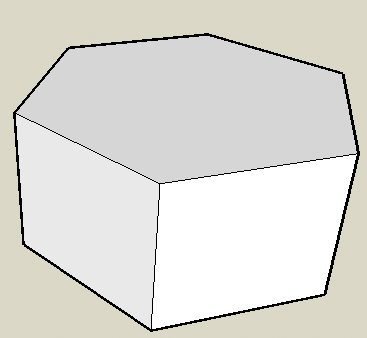SEARCH HOMEMath Central Quandaries & QueriesQuestion from Chris, a student: I am trying to figure out the volume of a regular octagonal prism, with side lengths of 4 inches. How do I do this?Chris,

Since you only give one length, 4 inches, I am going to assume that your regular octagonal prism is a right prism and its height is 4 inches.In that case the volume is the area of the octagon times the height of 4 inches. The area of a regular octagon can be found using our note on finding the area of a regular polygon.

PennyMath Central is supported by the University of Regina and The Pacific Institute for the Mathematical Sciences.